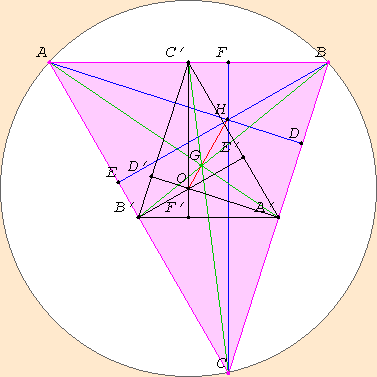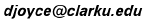# The Euler Line of a Triangle

It's amazing how much geometry there is in the lowly triangle! Consider the triangle ABC below (colored magenta). Several interesting points and lines can be constructed, and they all move as you drag about a vertex A, B, or C of the triangle.### The centroid G of the triangle

Along the sides of the triangle, you see the midpoints labelled A', B', and C'. (By the way, you can place your mouse cursor over the diagram and press the return key to lift the diagram off the page. You can move and resize the window that appears.) The midpoint of the side BC is A', the midpoint of the side CA is B', and the midpoint of the side AB is C'. A line connecting a vertex of the triangle to the midpoint of the opposite side is called a median of the triangle. The medians of this triangle are AA', BB', CC', and they’re colored green. Notice that they all meet at one point G in the triangle, also colored green. This point is called the centroid of the triangle. Other names for the centroid are the barycenter and the center of gravity of the triangle. If you make a real triangle out of cardboard, you can balance the triangle at this point.

It can be shown that the centroid trisects the medians, that is to say, the distance from a vertex to the centroid G is twice the distance from the centroid to the opposite side of the triangle. So, for instance, AG is twice A'G.

Incidentally, you can drag around other points besides the vertices A, B, and C. If you drag any other point, the figure is designed to swirl around the centroid G. An exception is G itself, and if you move it, the figure will slide along with it. Only moving A, B, or C will actually change the shape of the triangle.

### The circumcircle and the circumcenter O

You might have first noticed the circle on which the vertices of the triangle A, B, and C all lie. It is called the circumcircle of the triangle. Any three points, unless they lie on a straight line, determine a unique circle, this circumcircle. The center of this circle is called the circumcenter, and it’s denoted O in the figure. For acute triangles, the circumcenter O lies inside the triangle; for obtuse triangles, it lies outside the triangle; but for right triangles, it coincides with the midpoint of the hypotenuse.

As Euclid proved in Propsition IV.3 of his Elements, the circumcenter can be found as the intersection of the three perpendicular bisectors of the sides of the triangle. These are the lines perpendicular to the sides of the triangle passing through the midpoints of the sides. They’re labelled A'OD', B'OE', and C'OF', and they're colored black, as are the lines connecting the midpoints of the sides, A'B', B'C', and C'A'.

### The altitudes and the orthocenter H

There's yet another interesting “center” of the triangle, the orthocenter. An altitude of the triangle is a line drawn through a vertex perpendicular to the side of the triangle opposite the vertex. There are three altitudes: one is AD perpendicular to the side BC, the second is BE perpendicular to the side CA, and the third is CF perpendicular to the side AB. They're colored blue here. Note that when the triangle is obtuse, two of the altitudes lie outside the triangle, so they actually connect a vertex to the opposite side extended. In the case of a right triangle, two of the altitudes are actually sides of the triangle.

The altitudes of a triangle meet at a point, called the orthocenter, denoted here by H. For an acute triangle, the orthocenter lies inside the triangle; for an obtuse triangle, it lies outside the triangle; and for a right triangle, it coincides with the vertex at the right angle.

For fun, see what points and lines coincide for special triangles: isosceles triangles, right triangles, equilateral triangles, and right isosceles triangles.

### The Euler line OGH of the triangle

These three “centers” of the triangle lie on one straight line, called the Euler line. (“Euler” is pronounced something like “Oiler” in English.) Leonhard Euler (1707–1783) was a very prolific mathematician known for his discoveries in many branches of mathematics ranging from number theory to analysis to geometry.

It's surprising that these three points lie on a straight line. But you might see why from the picture.

Focus your attention on the centroid G. For each point, like A on one side of it, there is another, like A' on the other side of it but half as far away. On one side is B, the other B'; on one side C, the other C'. In fact, this correspondence sends the whole triangle ABC to the smaller, but similar, triangle A'B'C', called the medial triangle. The sides of the medial triangle A'B'C' are parallel and half the length of the sides of the original triangle ABC.

You can see from the figure that this correspondence sends the altitudes of the original triangle, which are AD, BE, and CF, to the altitudes of the medial triangle, which are A'D', B'E', and C'F'. Since the altitudes of the original triangle meet at the orthocenter H of the original triangle, the altitudes of the medial triangle will meet at its orthocenter H' which you can see in the figure is labelled O. Behold! This orthocenter O of the medial triangle is the circumcenter of the original triangle! Thus, this correspondence sends H to O, that is, H and O are on the opposite sides of the centroid G, and O is half as far away from G as H is.

This figure utilizes the Geometry Applet.

March, 1996.
David E. Joyce
Department of Mathematics and Computer Science
Clark University
Worcester, MA 01610The address of this file is http://aleph0.clarku.edu/~djoyce/java/Geometry/eulerline.html